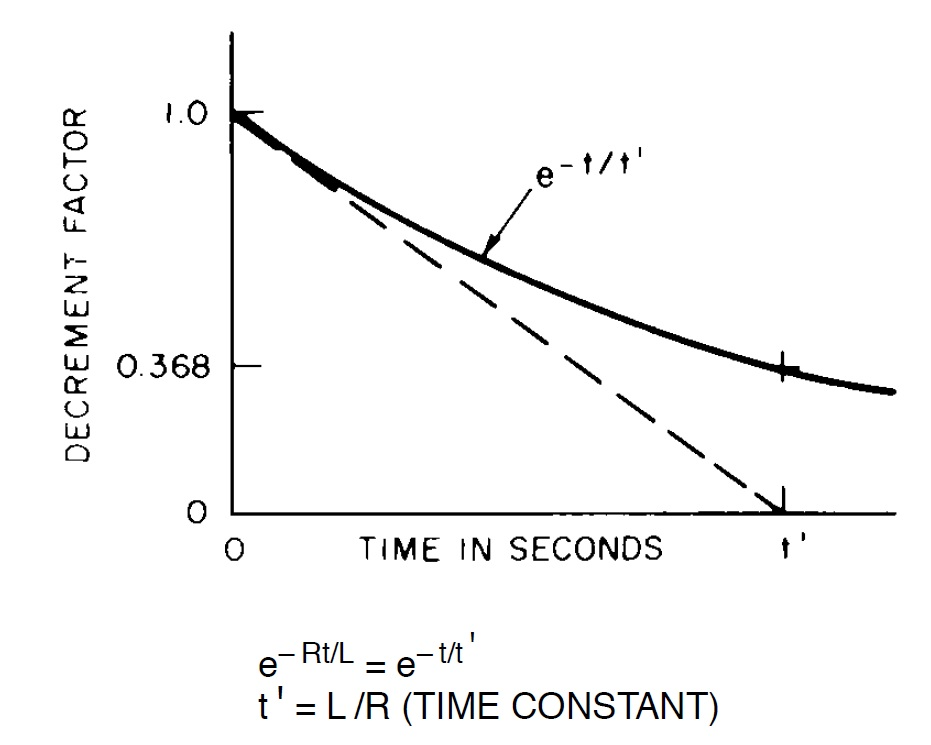# IEC 60909 vs ANSI/IEEE 141 Short-Circuit Current CalculationsShort-circuit calculation is one of the most important work to be undertaken in plant power distribution. A comprehensive, detailed and accurate short-circuit currents will be used in switchgear selection, protection settings and coordination.

Traditional short-circuit calculation primarily calculate the steady-state currents as they are significantly easier to calculate than transient or electromagnetic simulations. Steady-state currents however often fail to correctly calculate the AC and DC decay resulting from rotating machines which have notable contribution to the overall fault current at the fault. Steady-state calculations use only fixed impedances for rotating machines.

ANSI/IEEE 141 gives detailed guidelines on the short circuit calculations methodology and data requirements. The method presented in this standard is one of the simplest steady-state SCC in terms of the computational requirements. The machine impedances used for the calculation are mainly sub-transient reactances. The standard assumes unloaded network conditions, which means that the pre-fault load flow calculation for the network is not required.

The peak short-circuit current can be calculated multiplying the initial momentary SC current by a crest factor of 2.7. AC and DC decaying are encountered through use of the specified decrement factors. These factors depend on the X/R ratio of the fault impedance and the circuit breaker contact parting time. These will be different for near-to and far-from generators faults.

IEC 60909 short-circuit calculation is similar to the short-circuit current calculation ANSI/IEEE 141. The most important differences are the use of a voltage correction factors (c=1.1 for high-voltage networks) and impedance correction factors. The use of a voltage correction factor c increases the pre-fault magnitude of the voltage source applied to the network by 10%. This makes the fault current at least 10% higher and sometimes leads to more conservative results. The impedances of rotating machines are constant in this calculation. These constant impedances for synchronous machines are calculated using sub-transient impedances and the corresponding impedance correction factors. The impedances used for asynchronous (induction) motors are calculated using the locked rotor impedance values.

References:
1. IEEE Std 141-1993 IEEE Recommended Practice for Electric Power Distribution for Industrial Plants
2. CIRED - 19th International Conference on Electricity Distribution Vienna, 21-24 May 2007 Paper 0562